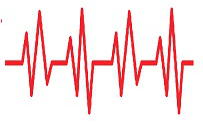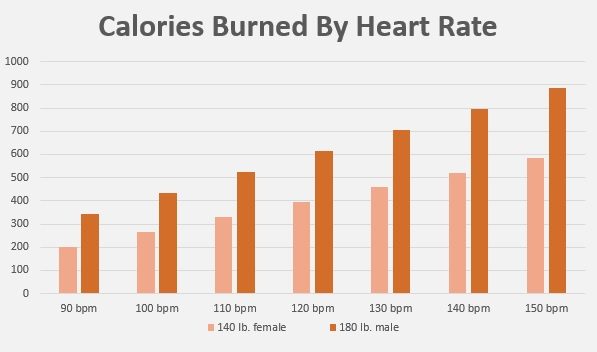## Calories Burned By Heart Rate

The number of calories you burn has a lot to do with your heart rate. In a study by the Journal of Sports and Sciences, a formula was developed based on weight, age, time, gender and heart rate. Use our tool to calculate how many calories you burn at a certain heart rate. This tool is only useful for athletes with a heart rate between 90 and 150. If you use a higher or lower heart rate, the calculation will be inaccurate.434

## About the formula we use for calories and heart rate

We use two different formulas for the calculation. One for women and the other for men. The formula can only be used if the heart rate is between 90 and 150 beats per minute. If you calculate with a heart rate lower than 90, the calculation will not be correct.

Men:
Calories per minute = (-55.0969 + (0.6309 * Heart rate) + (0.1988 * Weight) + (0.2017 * Age)) / 4.184

Women:
Calories per Minute = (-20.4022 + (0.4472 * Heart Rate) - (0.1263 * Weight) + (0.074 * Age)) / 4.184

Check out the table below to get an idea of how much calories burned as heart rate increases. We have counted on this with a person of 30 years. If you are under 30 years old, the numbers will be slightly lower. If you are older, the number of calories burned is higher. We did our calculations for a time period of 60 minutes. Use the tool above to calculate your personal calorie consumption at a certain heart rate.

Burned Calories and Heart Rate
140 lb. female 180 lb. male
90 bpm 201 344
100 bpm 266 434
110 bpm 330 525
120 bpm 394 615
130 bpm 458 706
140 bpm 522 797
150 bpm 586 886